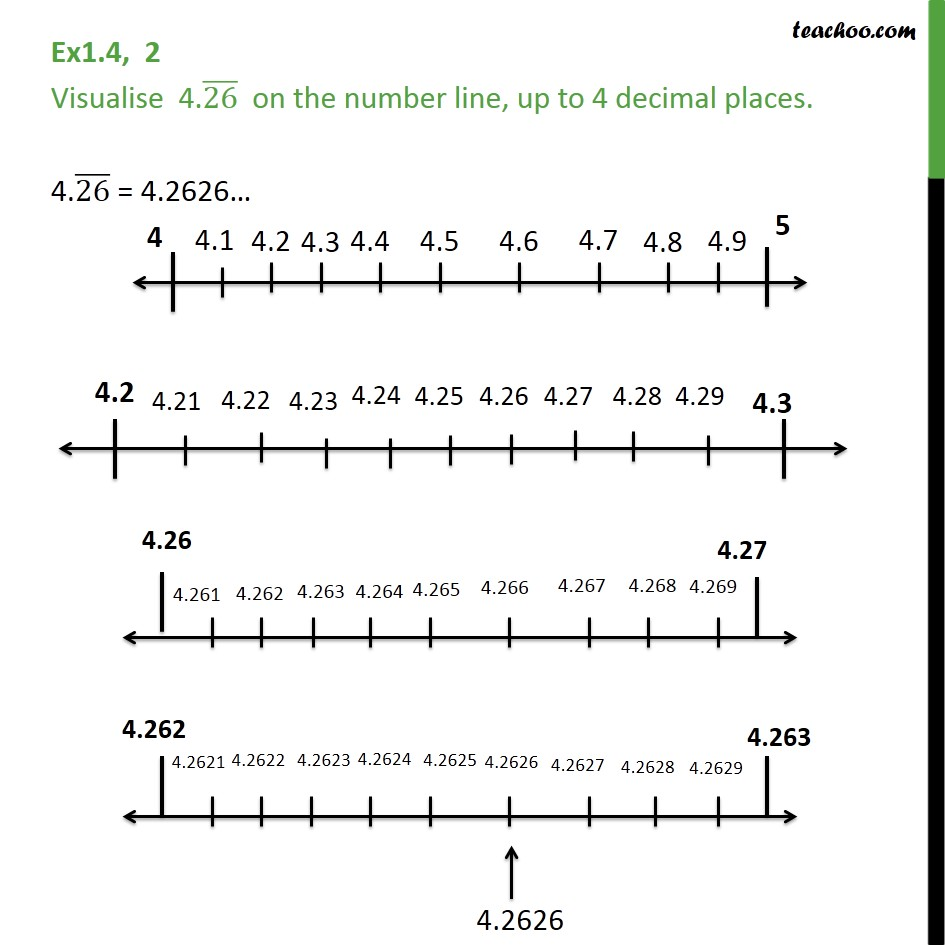Visualise Decimal Numbers on the number line

Chapter 1 Class 9 Number Systems
Serial order wiseLearn in your speed, with individual attention - Teachoo Maths 1-on-1 Class

### Transcript

Question 2 - Chapter 1 Class 9 Number Systems - NCERT Solutions Visualise 4.(26) ̅ on the number line, up to 4 decimal places. Since we have a bar on top of 26, 26 repeats So, writing upto 4 decimal places 4.(26) ̅ = 4.2626… Now, to mark 4.2626 on the number line, We follow these steps You can also check out the video The steps are 1. Draw number line from 4 to 5. 2. Since 4.2626 is greater than 4.2, but less than 4.3, We draw number line form 4.2 to 4.3 3. Now, Since 4.2626 is greater than 4.26, but less than 4.27, We draw number line form 4.26 to 4.27 4. Now, Since 4.2626 is greater than 4.262, but less than 4.263, We draw number line form 4.262 to 4.263 5. We can mark 4.2626